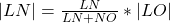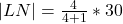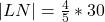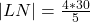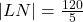## The points L, M, N and O all lie on the same line segment, in that order, such that the ratio of LM:MN:NOLM:MN:NO is equal to 3:1:2.3:1:2. I

Question

The points L, M, N and O all lie on the same line segment, in that order, such that the ratio of LM:MN:NOLM:MN:NO is equal to 3:1:2.3:1:2. If LO=30,LO=30, find LN.LN.

in progress 0
6 months 2021-07-18T23:13:10+00:00 1 Answers 19 views 0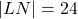Step-by-step explanation:

Given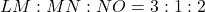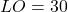Required

Find LN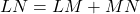From the ratio, LM = 3 and MN = 1

So: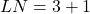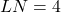LN and NO can be represented as: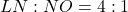The length of LN is then calculated as: